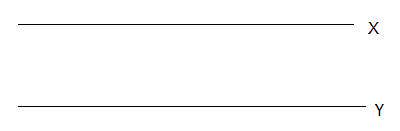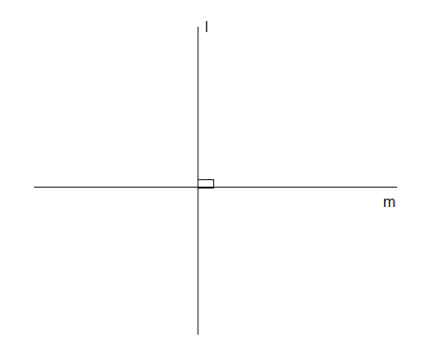# Geometry: Lines & Angles

Even though the quantitative section of the GRE syllabus does not include any trigonometry of 100 lines of proofs, it is important to remember that GRE is not void of any geometry. The geometry topics include many sections and various geometrical structures, one of which is lines and angles. The study of Geometry starts from grade school and the first concept emerging from it is the topic of points, lines and angles. Hence, without mastering this topic, all the concepts of the higher order geometrical figures will be a waste. GRE geometry questions always involve at least one question from this topic and with little dedication and practice you can conquer this topic with great ease.

Let us tackle these topics one at a time, starting from the concepts of points.

• Point: Any exact location or position on a plane surface is known as point. It is crucial to remember that point is a place, an address; it’s not a thing or name. It is usually represented by a dot and has no dimension. The naming of the points is usually done using uppercase alphabets.
• Collinear and Coplanar points: If the whole set of points lie on the same straight lines, these types of points are known as collinear. Whereas, if the complete set lies in a single plane, then it is known as coplanar points.
• Lines: Line is a combination of many points joined together and has their endpoints at infinity.
• Line Segment: It is a fragment of a line that has a fixed length with two endpoints.

Two important types of lines:

• Parallel lines: Two lines are said to be parallel if they lie in the same plane but never intersect one another. From the aspects of coordinate geometry, the slopes of parallel lines are always equal, though their intercepts may change. It is commonly represented with the symbol ||.• Perpendicular lines: Two lines are said to be perpendicular to one another if they make an angle of 90o at their point of intersection. It is represented by the symbol ┴.• Angles: When two lines share an endpoint (known as the vertex of the angle), their union point is the angle. The measurement of an angle is corresponded to the distance you need to rotate one line to reach the other line. The measurement scale of the angles is degree and the symbol used to represent degree is º. It should be noted that the symbol used for representing degree is not an exponent of 0, even though it does look pretty close. A complete rotation means 360o, hence it is quite evident that a circle make 360o, as well as the four angles formed from the intersecting lines add up to make 360 degrees.

BYJU’S will be glad to help you in your GRE preparation journey. You can ask for any assistance related to GRE from us by just giving a missed call at +918884544444, or you can drop an SMS. You can write to us at gre@byjus.com